# CUMPRINC Function

Calculates the cumulative principal paid on a loan taken or an investment made

## What is the CUMPRINC Function?

CUMPRINC Function is an Excel Financial function. This function helps calculate the cumulative principal amount paid on a loan, or the cumulative amount accrued by an investment. The function assumes a fixed interest rate and payment schedule. Most of the times, CUMPRINC and CUMIPNT are used together.

### Formula

=CUMPRINC(rate, nper, pv, start_period, end_period, type)

The CUMPRINC function uses the following arguments:

1. Rate (required argument) – This is the rate of interest per period.
2. NPER (required argument) – The total number of payment periods for which the loan or investment is to be paid.
3. PV (required argument) – This is the Present Value of the loan/Investment.
4. Start_period (required argument) – The number of the first period over which the interest is to be calculated. It needs to be an integer between 1 and the specified NPER.
5. End_period (required argument) – The last period over which interest is to be calculated. It must be an integer between 1 and the specified NPER.
6. Type (required argument) – This specifies the timing of the payment. It can be 0 or 1. It is an integer that specifies if the payment is made at the start of the period (0) or at the end of the period (1).

### How to use the CUMPRINC Function in Excel?

To understand the uses of the CUMPRINC function, let us consider a few examples:

#### Example 1

Let’s assume we took out a \$50,000 loan that needs to be paid back in full over 5 years. Interest charged by the bank is 5% and we are required to make monthly payments. First, we need to arrange the data in such a manner that it becomes easier to apply the formula and avoid errors.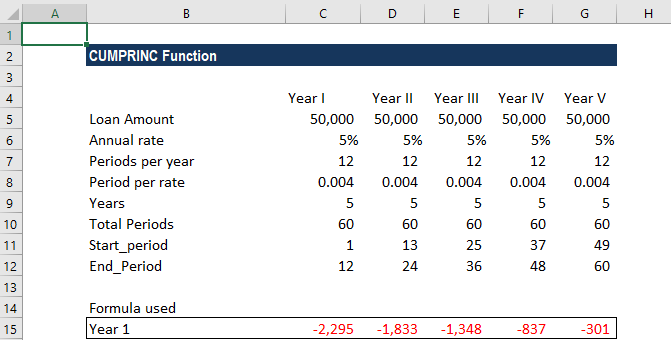We will do the same for each year of the loan. As seen in the screenshot above, the start and end periods change for each year. To avoid errors, we can use a formula. I used the following formula here for the start_period: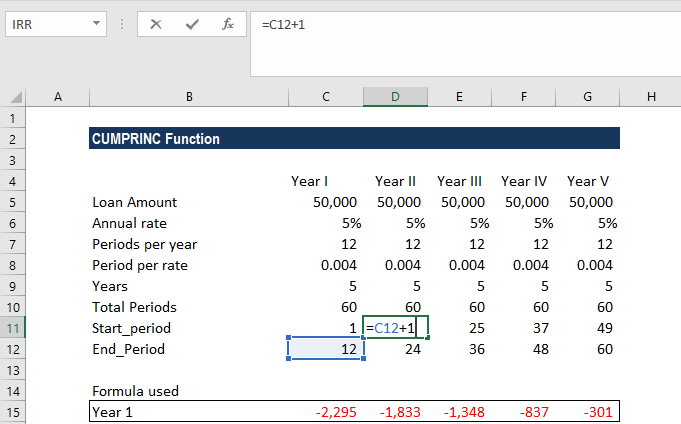For the end_period, I used the following formula: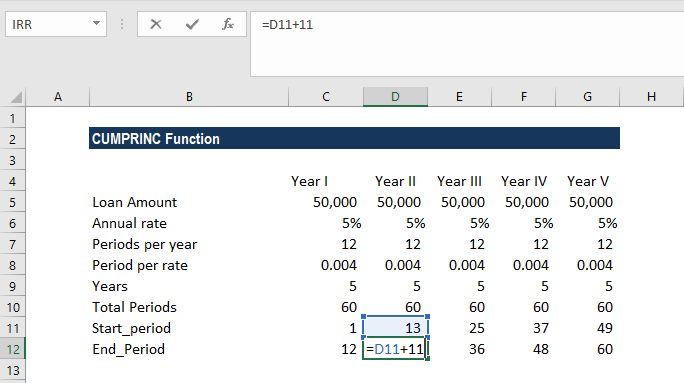As seen above, the interest rate charged is on a per annum basis so we need to convert it into a monthly rate (5%/12). This is the period rate in our table above.

Also, the total number of payments is 60, as we will be making monthly payments.

Let’s see how this formula can be used in the given scenario: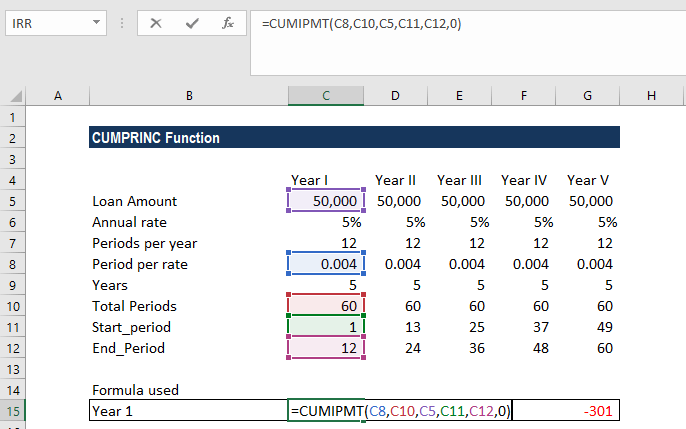We get the results below: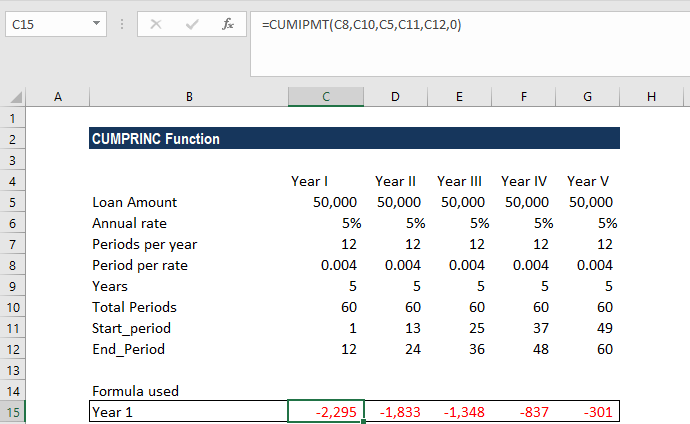The calculated interest payments are negative values as it represents outgoing payments for the person who took out the loan.

Often, this formula will give very low results. This generally happens when we forget to convert the annual interest rate or the number of periods to months or quarters as required.

To avoid errors, we need to make sure that we are consistent with the units used for specifying rate and nper.

#### Example 2

Using the same data as above, let us see how the results would change if we were required to make quarterly payments. Now, as we need to make quarterly payments, the number of periods per year will change to 4.

The table will look like below: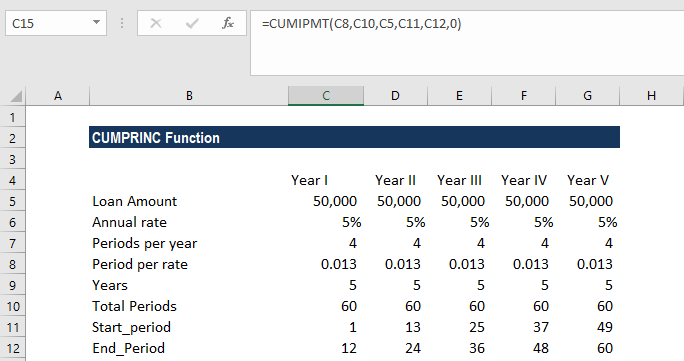The formula to use will be: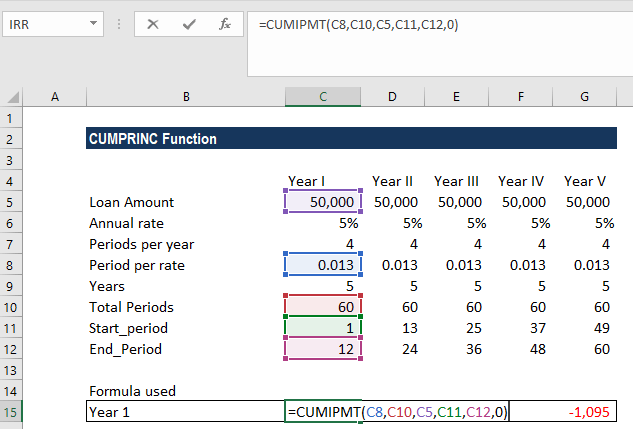We will get the following results: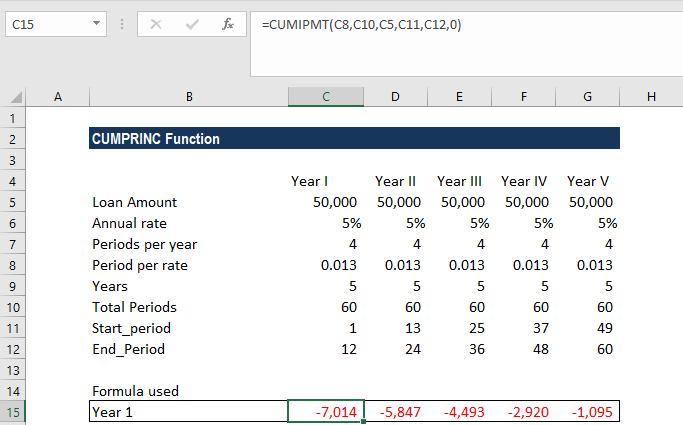### A few notes about the CUMPRINC Function

1. #NUM! error occurs in the following scenarios:
1. When the given start_period or end_period is less than or equal to zero.
2. When the given start_period is greater than the end_period.
3. When any of the given arguments – rate, nper, or PV – is less than or equal to zero.
4. The argument type is not equal to 0 or 1.
2. #VALUE! error – Occurs when any of the given arguments is a non-numeric value or is not recognized as a numeric value by Excel.

Thanks for reading CFI’s guide to important Excel functions! By taking the time to learn and master these functions, you’ll significantly speed up your financial analysis. To learn more, check out these additional CFI resources:

• Excel Functions for Finance